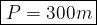## Find the perimeter of a football field which measures 90m by 60m

Question

Find the perimeter of a football field which measures 90m by 60m

in progress 0
2 months 2021-07-30T22:06:36+00:00 2 Answers 11 views 0

1. Hello!Use the following formula for the perimeter:

P = 2l + 2w, where:

l = length

w = width

Therefore:

P = 2(90) + 2(60)

Simplify:

P = 180 + 120 = 300 m